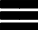Krivalar TutorialsKrivalar Tutorials

# Java BigInteger class

## BigInteger class

BigInteger is used to represent very large integer numbers when other numeric primitive datatype can be used. There is no limit on the size of the number that can be used.
Java BigInteger class has all the functionalities of 'int' primitive data type. Methods available in java.lang.Math for int primitive data type are also available for BigInteger.

BigInteger has several constructors. However the simplest of those are:

• BigInteger(byte[] val)
• BigInteger(String val)

## BigInteger - To determine whether a number is Probable Prime

``````
BigInteger(String.valueOf(i)).isProbablePrime(certainty)``````

## BigInteger - To determine the Greatest common denominator (GCD) of a number

GCD (Greatest common denominator ) is also called the highest common factor (HCF)

Method signature:
``````
BigInteger gcd(BigInteger val)``````
Usage Example:
``````
BigInteger num1 = new BigInteger("200501");
BigInteger num2 = new BigInteger("200501");
BigInteger gcdOfNum1andNum2= num1.gcd(num2);``````
In the above, the method gcd calculates the GCD of the two numbers num1 and num2.

### To determine reminder of a division

reminder() method returns a reminder of a division which can be positive, negative or zero

### To determine mod in modular arithmetic

mod() method returns only non-negative reminder